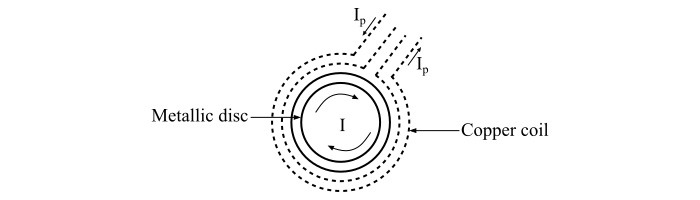# Induction Heating: Working Principle and Applications

Induction heating is the process of heating the materials which are electrically conductive by electromagnetic induction like metals.

## Working Principle of Induction Heating

Induction heating makes use of the induced current by the electromagnetic induction in the charge to be heated. The induction heating works on the principle of transformer action, in which the primary winding which is supplied from an AC source is magnetically coupled to the charge, to be heated, which acts as a short-circuited secondary of a single turn.

When the AC supply is connected to the primary winding, it induces currents in the charge. As the secondary, i.e., the charge has some resistance, thus the induced currents in the charge heats it up.

## Factors Affecting Induction Heating

In order to analyze the factors affecting the induction heating process, let us consider a circular disc which has to be heated. The disc carrying a current of I amperes at a frequency of f Hz; refer the figure.Now, the following factors affect the heat developed in the disc −

• Primary coil current

• Number of turns in the primary coil

• Frequency of the supply source

• The magnetic coupling between the coil and the disc

• The electrical resistivity of the disc.

If the charge to be heated is of non-magnetic material, then the heat developed is due to eddy current loss, while, if the charge is of magnetic material, the heat will be developed due to both hysteresis loss and eddy current loss. Both the hysteresis and eddy current losses depend upon the supply frequency.

Therefore, the depth (d) of the penetration of induced currents into the disc is given by,

$$\mathrm{\mathit{d}\:=\:\frac{1}{2\pi}\sqrt{\frac{\rho \times 10^{\mathrm{9}}}{\mu {\mathit{f}}}}\:\mathit{cm}}$$

$$\mathrm{\therefore \mathit{d}\propto \frac{1}{\sqrt{\mathit{f}}}}$$

Where,

• ρ is the resistivity of the disc material in ω-cm.

• f is the supply frequency in Hz.

• μ is the permeability of the charge material.

## Applications of Induction Heating

The induction heating is used in the following applications −

• Inductive heating is used for surface heating, melting and soldering, etc.

• Inductive heating is also used for heating of liquid conductors and gaseous conductors.

• In semiconductor industries, the inductive heating is used for heating of silicon.

• Inductive heating is used in inductive furnaces for heating the metals to their melting point.

• The induction stoves used in kitchen works on the principle of inductive heating.

• As the induction heating is contactless heating process. Therefore, induction heating is used in vacuum furnaces for making specialized steel and alloys that would get oxidized when heated in the presence of oxygen.

• The induction heating is used in plastic injection modeling machines.

• The induction heating is also used for tamper resistant cap sealing on bottles and pharmaceuticals.

• Induction heating is used for welding of metals and sometimes plastics when they are doped with ferromagnetic ceramics.